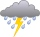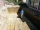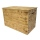# Sugar - cuboid

Pejko received from his master cuboid composed of identical sugar cubes with count between 1000 and 2000. The Pejko eat sugar cubes in layers.
The first day eat one layer from the front, second day one layer from right, the third day one layer above. Yet in those three layers were each the same number of cubes. Find out how many cubes had original cuboid. Determine all possibilities.

Correct result:

V1 =  1320
V2 =  1716

#### Solution:

PHP program:

for($a=1;$a<1000; $a++) { for($b=1; $b<1000;$b++)
{
for($c=1;$c<1000; $c++) {$V = $a*$b*$c; if($V > 2000 || $V<1000) { continue; } if($a*$b == ($c-1)*$b && ($c-1)*$b == ($a-1)*($c-1)) { echo "$V = $a *$b * \$c <br>";
}
}
}
}Our examples were largely sent or created by pupils and students themselves. Therefore, we would be pleased if you could send us any errors you found, spelling mistakes, or rephasing the example. Thank you!

Please write to us with your comment on the math problem or ask something. Thank you for helping each other - students, teachers, parents, and problem authors.Tips to related online calculators
Do you solve Diofant problems and looking for a calculator of Diofant integer equations?
Tip: Our volume units converter will help you with the conversion of volume units.

## Next similar math problems:

• Identical cubesFrom the smallest number of identical cubes whose edge length is expressed by a natural number, can we build a block with dimensions 12dm x 16dm x 20dm?
• Four prismsQuestion No. 1: The prism has the dimensions a = 2.5 cm, b = 100 mm, c = 12 cm. What is its volume? a) 3000 cm2 b) 300 cm2 c) 3000 cm3 d) 300 cm3 Question No.2: The base of the prism is a rhombus with a side length of 30 cm and a height of 27 cm. The heig
• Aquarium heightHow high does the water in the aquarium reach, if there are 36 liters of water in it? The length of the aquarium is 60 cm and the width is 4 dm.
• Water tank300hl of water was filled into the tank 12 m long and 6 m wide. How high does it reach?
• Block or cuboidThe wall diagonals of the block have sizes of √29cm, √34cm, √13cm. Calculate the surface and volume of the block.
• Two rectangular boxesTwo rectangular boxes with dimensions of 5 cm, 8 cm, 10 cm, and 5 cm, 12 cm, 1 dm are to be replaced by a single cube box of the same cubic volume. Calculate its surface.
• Cuboid and ratioFind the dimensions of a cuboid having a volume of 810 cm3 if the lengths of its edges coming from the same vertex are in ratio 2: 3: 5Calculate the volume (V) and the surface (S) of a regular quadrilateral prism whose height is 28.6 cm and the deviation of the body diagonal from the base plane is 50°.
• Flower boxesHow many m2 of 10mm thick boards are needed to make 12 flower boxes? The dimensions of the box are 180,150 and 1500 mm.
• RainGarden shape of a rectangle measuring 15 m and 20 m rained water up to 3 mm. How many liters of water rained on the garden?
• The excavationThe excavation for the city sewer was 38 m long, 2.2 m wide, and 3 m deep. a) How many cubic meters of soil were dredged b) How many journeys had to be made by one car when removing the soil, if they loaded an average of 4.5 m of cubic soil on each journe
• Milk cartonsHow much paper do we need for 12 tetra pack with dimensions 6 cm, 11 cm and 20 cm? Will 1 liter of milk fit in the box?
• Cuboid edgesCalculate the volume and surface of a cuboid whose edge lengths are in the ratio 2: 3: 4 and the longest edge measures 10cm.
• Cuboid to cubeA cuboid with dimensions of 9 cm, 6 cm, and 4 cm has the same volume as a cube. Calculate the surface of this cube.
• Surface and volumeFind the surface and volume of a cuboid whose dimensions are 1 m, 50 cm, and 6 dm.
• Wooden boxThe block-shaped box was placed on the ground, leaving a rectangular print with dimensions of 3 m and 2 m. When flipped over to another wall, a print with dimensions of 0.5 m and 3 m remained in the sand. What is the volume of the wooden box?
• Volume and surface areaFind the volume and surface of a wooden block with dimensions: a = 8 cm, b = 10 cm, c = 16 cm.